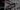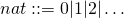# Parsers in Scala built upon existing abstractions

After some initial struggles, the chapter Functional Parsers from the great book Programming in Haskell by Graham Hutton, where a basic parser library is built from scratch, significantly helped me to finally understand the core ideas of parser combinators and how to apply them to other programming languages other than Haskell as well.

While I recently revisited the material and started to port the examples to Scala I wasn't able to define a proper monad instance for the type Parser[A].

The type Parser[A] alias was defined like this:

type Parser[A] = String => Option[(A, String)]
// defined type alias Parser


To test the monad laws with discipline I had to provide an instance of Eq[Parser[A]]. Because Parser[A] is a function, equality could only be approximated by showing degrees of function equivalence, which is not a trivial task.

Also the implementation of tailRecM was challenging. (I couldn't figure it out.)

## Using existing abstractions

Functional programming offers a lot of abstractions provided either by the core languages or by external libraries suited to solve every day low-level (domain agnostic) problems.

A good example is the Option type that models the potential absence of a value. Without Option we would be forced to redundantly reimplement low-level imperative handling of nulls and null reference exceptions everywhere.

Apparently it is a good practice to refine our types so that they can be applied to certain categories of problems that have already been solved. Then we can reuse existing abstractions that are battle tested just such as Option.

It turns out that the Parser type looks very similar to the state monad which is a monad instance on the function S => (S, A) where S represents the state. In the case of a parser the input state represents the input string and the output state represents the remaining unparsed string whereas A represents the result type of the parser.

When we now take into account that parsers might fail we can stack the State on top of an Option with the help of monad transformers.

Let's see if we've found an appropriate existing abstraction for parsers (disregarding stack-safety for now) by trying to implement a parser library on top of it.

We can now define the Parser[A] type in terms of StateT and Option from Cats like this:

import cats.data.StateT._
import cats.data.StateT
import cats.implicits._

type Parser[A] = StateT[Option, String, A]
// defined type alias Parser


## Implementing the parser library

E.g. we don't have to implement combinators for sequencing parsing operations. This is already taken care of by the combined monad instances of State and Option with the flatMap operation.

Because we get a Functor instance for free we can map over inner values of parsers.

Also the parser that always succeeds is just pure provided by the Applicative instance of the monad and the parser that always fails is raiseError provided by ApplicativeError:

"42".pure[Parser]
// res0: Parser[String] = cats.data.IndexedStateT@6f2c0142

().raiseError[Parser, Nothing]
// res1: Parser[Nothing] = cats.data.IndexedStateT@2cb7bb20


The first real parser we will implement is item which parses whatever next character there is. Even though, it's possible to do this a little more concise, we will use the idiomatic get and modify functions from StateT:

val item: Parser[Char] =
for {
input <- get[Option, String]
_ <- if (input.nonEmpty)
modify[Option, String](_.tail)
else
().raiseError[Parser, Nothing]


Now let's define a function to create a parser for single characters that satisfy a given predicate:

def sat(p: Char => Boolean): Parser[Char] =
for {
c <- item
_ <- if (p(c)) c.pure[Parser]
else ().raiseError[Parser, Nothing]
} yield c


This allows us to create some more primitive parsers:

val digit: Parser[Char] = sat(_.isDigit)

val lower: Parser[Char] = sat(_.isLower)

val upper: Parser[Char] = sat(_.isUpper)

val letter: Parser[Char] = sat(_.isLetter)

val alphaNum: Parser[Char] = sat(_.isLetterOrDigit)

def char(c: Char): Parser[Char] = sat(_ == c)


A parser for string literals can either be defined recursively or like this:

def string(str: String): Parser[String] =
str.map(char).toList.sequence.map(_.mkString)


Next we need a combinator that applies a first parser and if it fails then applies a second parser. There is already such a combinator for StateT comming from SemigroupK called combineK or <+>:

val p = string("hi") <+> string("hello")
// p: Parser[String] = cats.data.IndexedStateT@1c7a25f

p.run("hello world")
// res8: Option[(String, String)] = Some(( world,hello))


With the help of <+> we can define two mutually recursive parsers many and many1 that repeatedly apply parsers until they fail and return lists of results of 0 to n or 1 to n elements:

object Many { // only needed for tut
def many[A](p: Parser[A]): Parser[List[A]] =
many1(p) <+> List.empty[A].pure[Parser]

def many1[A](p: Parser[A]): Parser[List[A]] =
for {
tail <- many(p)
}

import Many._


The parsers and combinators that we have implemented so far together with a parser for zero to many white spaces now can be used to create parsers for identifiers, natural numbers and symbols:

val ident: Parser[String] =
(lower, many(alphaNum)).mapN(_ :: _).map(_.mkString)

val nat: Parser[Int] =
many1(digit).map(_.mkString.toInt)

val space: Parser[Unit] =
many(sat(_.isWhitespace)).map(_ => ())

def token[A](p: Parser[A]): Parser[A] =
space *> p <* space

val identifier: Parser[String] = token(ident)
val natural: Parser[Int] = token(nat)
def symbol(s: String): Parser[String] = token(string(s))


token is implemented with *> and <*. These operators come from the Apply typeclass. They compose two values and discard one of them. In this case the spaces around the token are discarded while the token is kept.

## Building a parser for arithmetic expressions

We have now everything we need to compose more complex parsers, e.g. to parse and evaluate arithmetic expressions like 2 * 3 + 4 * (1 + 5) according to the following grammar where the symboldenotes the empty string:Here is a direct translation of the grammar to our parser primitives:

object Arithmetics {
lazy val expr: Parser[Int] =
for {
t <- term
res <- (for {
_ <- symbol("+")
e <- expr
} yield t + e) <+> t.pure[Parser]
} yield res

lazy val term: Parser[Int] =
for {
f <- factor
res <- (for {
_ <- symbol("*")
t <- term
} yield f * t) <+> f.pure[Parser]
} yield res

lazy val factor: Parser[Int] =
(for {
_ <- symbol("(")
e <- expr
_ <- symbol(")")
} yield e) <+> natural
}

import Arithmetics._


Finally we define a function eval to evaluate expressions to either an Int value or an error if the expression is invalid or if there is any unconsumed input left:

def eval(input: String): Either[String, Int] =
expr.run(input) match {
case Some(("", n))  => Right(n)
case Some((out, _)) => Left(s"unconsumed input: \$out")
case None           => Left("invalid input")
}


Let's check some examples:

eval("2*(3+4)")
// res12: Either[String,Int] = Right(14)

eval("2 * 3 +  4")
// res13: Either[String,Int] = Right(10)

eval("2*(     3+ 4)  ")
// res14: Either[String,Int] = Right(14)

eval("2*3")
// res15: Either[String,Int] = Right(6)

eval(" (( 1 ))*( 2+( ( (   3) ) )* (4+(  ((5))+ 6))*( ((7*8   ))) +9)")
// res16: Either[String,Int] = Right(2531)


## Conclusion

I think this is pretty cool.

Out of nothing but few a primitives we have created a library of parsers and combinators of significantly less than 100 lines of code that allowed us to build parsers for relatively complex arithmetic expressions.

Applying battle tested existing abstractions provided a lot of built in functionalities that we didn't have to implement or test ourselves. This also reduced the amount of boiler-plate code by a great deal.

Note that here are a lot more features and compositional capabilities that we get from the abstraction that we chose that we didn't even use in these examples.

I'll give one more example, though, which I discovered with some help from the Cats Gitter channel (thanks to Luka Jacobowitz and Fabio Labella). Let's say we want to implement a parser by providing a list of parsers and try to apply each one, until one of them succeeds, semantically providing a list of alternatives. We get this for free:

val ps =
List[Parser[Char]](
char('a'),
char('b'),
lower,
digit)

ps.foldK.run("foo")
// res19: Option[(String, Char)] = Some((oo,f))


Here is the primary take away from this post: When you are dealing with specific recurring problems of implementation details it is very likely that these problems have been solved before. Try to modify and refine your model and your types in such a way that you can reuse and benefit from existing abstraction.

All examples are build with tut.